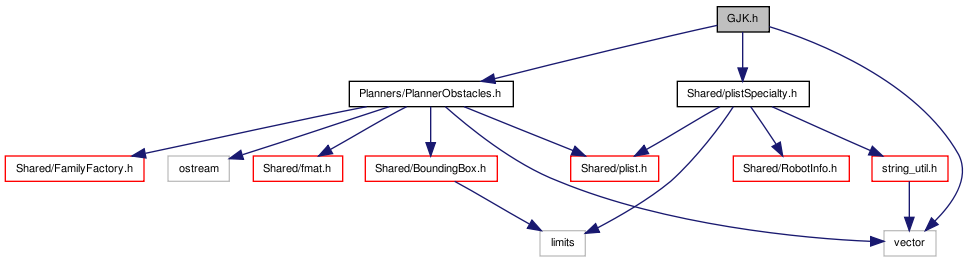# GJK.h File Reference

`#include "Planners/PlannerObstacles.h"`
`#include "Shared/plistSpecialty.h"`
`#include <vector>`
Include dependency graph for GJK.h:Go to the source code of this file.

namespace  GJK

## Functions

template<size_t N>
fmat::Column< N > GJK::tripleProduct (const fmat::Column< N > &a, const fmat::Column< N > &b, const fmat::Column< N > &c)
Returns (a x b) x c = b * (ac) - a * (bc).
template<size_t N>
bool GJK::processLine (std::vector< fmat::Column< N > > &simplex, fmat::Column< N > &direction)
template<size_t N>
bool GJK::processTriangle (std::vector< fmat::Column< N > > &simplex, fmat::Column< N > &direction)
template<size_t N>
bool GJK::processTetrahedron (std::vector< fmat::Column< N > > &simplex, fmat::Column< N > &direction)
template<size_t N>
bool GJK::processSimplex (std::vector< fmat::Column< N > > &simplex, fmat::Column< N > &direction)
template<size_t N>
fmat::Column< N > GJK::getSupport (const PlannerObstacle< N > *obs1, const PlannerObstacle< N > *obs2, const fmat::Column< N > &direction)
template<size_t N>
bool GJK::collides (const PlannerObstacle< N > *obs1, const PlannerObstacle< N > *obs2)

 Tekkotsu v5.1CVS Generated Mon May 9 04:58:56 2016 by Doxygen 1.6.3